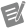# Accuracy

NI Digital Multimeters Help (NI-DMM 18.1)

Edition Date: March 2018

Part Number: 370384V-01

»View Product InfoDownload Help (Windows Only)

A reading from a DMM can differ from the actual input. Accuracy represents the uncertainty of a given measurement and can be defined in terms of the deviation from an ideal transfer function, as follows:

y = mx + b

where

x is the input

m is the ideal gain of a system

b is the offset

Applying this example to a DMM signal measurement, y is the reading obtained from the DMM with x as the input, and b is an offset error that you may be able to null before the measurement is performed. If m is 1, the output measurement is equal to the input. If m is 1.000001, then the error from the ideal is 1 ppm or 0.0001%.

The following table shows some ppm to percent conversions.

ppm Percent
1 0.0001
10 0.001
100 0.01
1,000 0.1
10,000 1

Most high-resolution, high-accuracy DMMs describe accuracy in units of ppm (DC functions) and percentage (AC functions). Therefore, DC and AC accuracy specifications commonly appear as ±(ppm of reading + ppm of range) or ±(% of reading + % of range), respectively. The reading component is the deviation from the ideal m, and the range component is the deviation from the ideal b, which is zero. The b errors are most commonly referred to as offset errors.

Hence, accuracy is often expressed as:

or

or

±(ppm of reading + ppm of range)Note  To determine which method is used, refer to the specifications document included with the DMM you are using.

For example, assume a DMM set to the 10 V range is operating 90 days after calibration at 23 ºC ±5 ºC and is expecting a 7 V signal. The DC accuracy specifications for these conditions state ±(20 ppm of reading + 6 ppm of range). To determine the accuracy of the measurement under these conditions, use the following formula:

Accuracy = ±(ppm of reading + ppm of range) = ±(20 ppm of 7 V + 6 ppm of 10 V) = ±((7 V(20/1,000,000) + (10 V(6/1,000,000)) = ±200 µV

Therefore, the reading should be within ±200 µV of the actual input voltage.Note  Temperature can have a significant impact on the accuracy of a DMM and is a common problem for precision measurements. The temperature coefficient, or tempco, expresses the error caused by temperature. Errors are calculated as ±(ppm of reading + ppm of range)/ºC or ±(% of reading + % of range)/ºC. Refer to Self-Calibration for examples of these calculations.